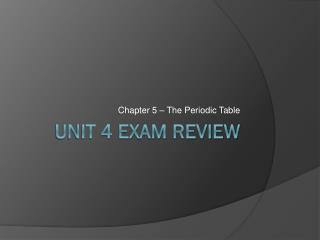Download PresentationUnit 4 Exam Review

# Unit 4 Exam Review - PowerPoint PPT Presentation

Chapter 5 – The Periodic Table. Unit 4 Exam Review. Question 1. The number 97 represents:. Question 1. The number 97 represents:. Question 2. What do the letters Bk represent in the above figure?. Question 2. What do the letters Bk represent in the above figure?. Question 3.I am the owner, or an agent authorized to act on behalf of the owner, of the copyrighted work described.
Download Presentation## Unit 4 Exam Review

Download Policy: Content on the Website is provided to you AS IS for your information and personal use and may not be sold / licensed / shared on other websites without getting consent from its author.While downloading, if for some reason you are not able to download a presentation, the publisher may have deleted the file from their server.

- - - - - - - - - - - - - - - - - - - - - - - - - - E N D - - - - - - - - - - - - - - - - - - - - - - - - - -
Presentation Transcript
1. Chapter 5 – The Periodic Table Unit 4 Exam Review

2. Question 1 The number 97 represents:

3. Question 1 The number 97 represents:

4. Question 2 • What do the letters Bk represent in the above figure?

5. Question 2 • What do the letters Bk represent in the above figure?

6. Question 3 The number 247.00 in the above figure represents:

7. Question 3 The number 247.00 in the above figure represents:

8. Question 4 The atomic mass of an element is:

9. Question 4 The atomic mass of an element is:

10. Question 5 The unit for atomic mass is the:

11. Question 5 The unit for atomic mass is the:

12. Question 6 Mendeleev arranged the known chemical elements in a table according to increasing

13. Question 6 Mendeleev arranged the known chemical elements in a table according to increasing

14. Question 7 When moving from left to right across a row of the periodic table, which of the following values increases by exactly one from element to element?

15. Question 7 When moving from left to right across a row of the periodic table, which of the following values increases by exactly one from element to element?

16. Question 8 In the periodic table, the periods are the

17. Question 8 In the periodic table, the periods are the

18. Question 9 The figure below shows a portion of a blank periodic table. Identify the segments labeled A and B.

19. Question 9 The figure below shows a portion of a blank periodic table. Identify the segments labeled A and B.

20. Question 10 Region 1 in the periodic table above is known as:

21. Question 10 Region 1 in the periodic table above is known as:

22. Question 11 Region 2 in the periodic table above is known as:

23. Question 11 Region 2 in the periodic table above is known as:

24. Question 12 Region 3 in the periodic table above is known as:

25. Question 12 Region 3 in the periodic table above is known as:

26. Question 13 Region A in the periodic table above contains

27. Question 13 Region A in the periodic table above contains

28. Question 14 Region B in the periodic table above contains

29. Question 14 Region B in the periodic table above contains

30. Question 15 Region C in the periodic table above contains

31. Question 15 Region C in the periodic table above contains

32. Question 16 How many valence electrons do members of the boron family have?

33. Question 16 How many valence electrons do members of the boron family have?

34. Question 17 The elements located in group 7A of the periodic table (also known as group 17) are called the:

35. Question 17 The elements located in group 7A of the periodic table (also known as group 17) are called the:

36. Question 18 The elements in group 8A (also known as column 18) of the periodic table are called:

37. Question 18 The elements in group 8A (also known as column 18) of the periodic table are called:

38. Question 19 Which of the following categories includes the majority of the elements?

39. Question 19 Which of the following categories includes the majority of the elements?

40. Question 20 Which list of elements contains only metals?

41. Question 20 Which list of elements contains only metals?

42. Question 21 Which of the following is a transition metal?

43. Question 21 Which of the following is a transition metal?

44. Question 22 Malleability and ductility are characteristics of:

45. Question 22 Malleability and ductility are characteristics of:

46. Question 23 Elements that share properties of both metals and nonmetals are called

47. Question 23 Elements that share properties of both metals and nonmetals are called

48. Question 24 Nonmetals tend to be

49. Question 24 Nonmetals tend to be

50. Question 25 Elements that belong to the same group have the same number of